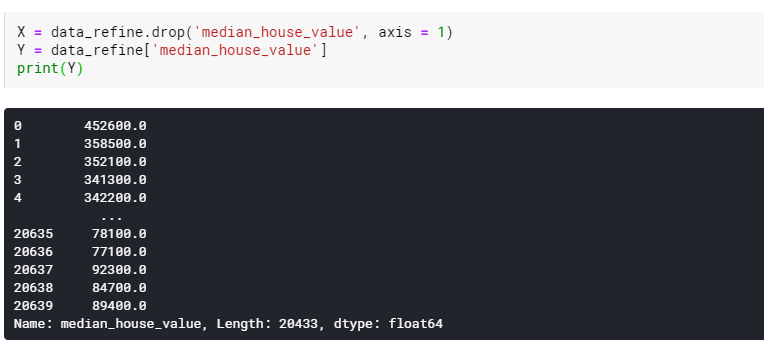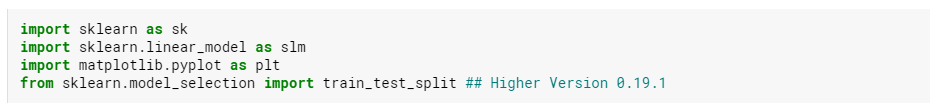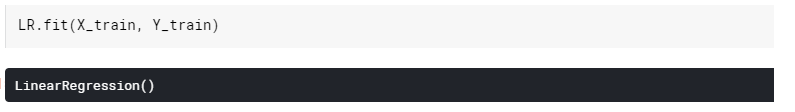Search

# Intro to Machine Learning using My First Machine Learning Model in PythonMachine learning is enabling computers to do tasks that have only been done by people, until now. From driving cars to recognizing speech and translating them, machine learning is creating a storm in the field of Artificial Intelligence(AI) by helping software predict the unpredictable real world.

So, What is Machine Learning?

Machine Learning is the process of teaching a computer system to make predictions using the past data that is fed to it. It is basically to train the computer with the help of past data to predict future data.

Those predictions could be as simple as finding out whether the animal in a photo is a cat or a dog, to something like recognizing speech accurately to generate captions for a website or to run a video or music.

Types of Machine Learning

Machine learning is generally split into two main categories: Supervised and Unsupervised learning.

Supervised Learning is an approach where machines are taught by example. The machines are trained with large amounts of data, so that the system would learn to recognize the pattern and can be used to identify and distinguish data based on the data it got trained on.

On the other hand, Unsupervised Learning uses algorithms to identify patterns in data sets containing data points that are neither classified nor labelled. The algorithms analyze the underlying structure of the data sets by extracting useful information or features from them and categorize the data based on the analysis.

Let’s see how to create a machine learning model using the supervised learning approach.

Step 1: Familiarize with data

The first step in any machine learning project is familiarize yourself with the data. Let’s use the Pandas library for this. Pandas is the primary tool that data scientists use for exploring and manipulating data.The most important part of the Pandas library is the DataFrame. A DataFrame is similar to a table which holds the data. This is similar to a table in a SQL database. Pandas has powerful methods for manipulating the data in the DataFrame.

For example, let us take the data of California housing prices which is at the file path ../input/california-housing-prices/housing.csv.

Let’s load and explore the data with the following commands:Step 2: Selecting Data for Modeling

After studying the data in the DataFrame, we can see that it has 10 columns and out of 10, 9 have numeric data and the “Ocean proximity” column has string type data. To build any model, we need only numeric data. So, let’s drop the “Ocean Proximity” column.Then , drop the rows that has null values as shown below:Step 3: Selecting The Prediction Target (Y) and features (X)

Next step is to choose the prediction target (Y) which in this case is “median_house_value” column. So, assign Y as “median_house_value”.

The remaining features will be X . So, let’s remove “median_house_value” column from the dataframe and assign others to X as shown below:Step 4: Building the model

We will use the scikit-learn library to create the models. This library is written as sklearn in the code. Scikit-learn is the most popular library for modeling the types of data stored in DataFrames.

The steps to building and using a model are:

• Define: What type of model will it be? Linear regression model? Some other type of model?

• Fit: Capture patterns from provided data. This is the heart of modeling.

• Predict: predict the target

• Evaluate: Determine how accurate the model's predictions are.

Let’s now define linear regression model with scikit-learn(sklearn) and fitting it with the features and target variable and get the predicted value of “median_house_value”.

Import the following libraries to use scikit-learn(sklearn) :Create a variable for Linear regression model. And also use the train_test_split function to split the data into training and testing data. Here I have used 25% of data for testing and remaining 75% for training the model.Step 5: Fit the model:

Now with the training data, fit the linear regression model.Once done, use the predict function to predict the housing values using X test values.

We can then use the score function to get the accuracy of the predicted values by the model as shown below:You can see that the model has predicted around 66% accurately.

Step 6: Plot the graph

Now let’s try to plot the graph using the X_test values and the predict values(output) as shown below:We now have a fitted model that we can use to make predictions.

In practice, we will want to make predictions for new houses coming on the market rather than the houses we already have prices for.

I have just shown here how to fit a linear regression model on a dataset and use it to predict the housing prices. We can also fit the same data to a decision tree or support vector machines and compare which model predicts better.

Hope this will help people who are trying to build their first machine learning linear regression model.

Happy Modelling!

Reference: Kaggle courses - https://www.kaggle.com/learn/intro-to-machine-learning

See All